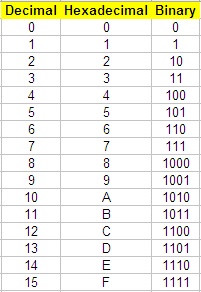# Hex to binary: two solutions in Matlab

 To convert a value from hex to binary, we first need to know what a hexadecimal number is. A major numbering system used in digital systems is the hex system, also known as base 16. In this system, the numbers are counted from 0 to F. In base 16 we need 16 different symbols, decimal numbers 10 through 15 are represented by letters A through F, respectively.

The  binary system (base 2) has only two symbols, '0' and '1'.

The following table shows the meaning of all the symbols in the hexadecimal  numbering system and their conversion to decimal or binary equivalents.To convert a value from hex to binary, you merely translate each hex symbol to the equivalent 4-bit binary group. For example, the hex number A5A5 translates into the binary 1010010110100101 equivalent.

### Solution 1. Conversion from hex to decimal, and then from decimal to binary

In Matlab, we can go from hexadecimal to decimal, and then, from decimal to binary. We can embed one instruction into the other, like this:

bin_str = dec2bin(hex2dec(hex_str))

So, we can use this concept, like this:

hex_str = 'AF5'
bin_str = dec2bin(hex2dec(hex_str))

bin_str = 101011110101

It’s important to remember that both binary numbers and hexadecimal ones are treated as strings in Matlab.

### Solution 2. Conversion using a Switch-Case structure

Now, let’s say that we want to explore how the hex characters are separated to form the binary symbols and how we can manipulate our own strings (binary and hexadecimal).

We can develop a function to translate the table shown before. Our proposed method uses a switch-case structure.

% Hex to binary conversion
function b = h2b(h)
switch h

case {'0'}
b =
'0000';

case {'1'}
b =
'0001';

case {'2'}
b =
'0010';

case {'3'}
b =
'0011';

case {'4'}
b =
'0100';

case {'5'}
b =
'0101';

case {'6'}
b =
'0110';

case {'7'}
b =
'0111';

case {'8'}
b =
'1000';

case {'9'}
b =
'1001';

case {'A', 'a'}
b =
'1010';

case {'B', 'b'}
b =
'1011';

case {'C', 'c'}
b =
'1100';

case {'D', 'd'}
b =
'1101';

case {'E', 'e'}
b =
'1110';

case {'F', 'f'}
b =
'1111';
end

Now, we have to call the function for every hex character in the string, to convert each to a 4-bit binary number. One possible solution to separate the hex characters into 4-bit groups is shown here:

hex_str = input(
n = length(hex_str);

bin_str = '';
for h = 1 : n
bin_str = [bin_str h2b(hex_str(h))
];
end
bin_str

Let’s try this routine.

bin_str = 1010111101010000